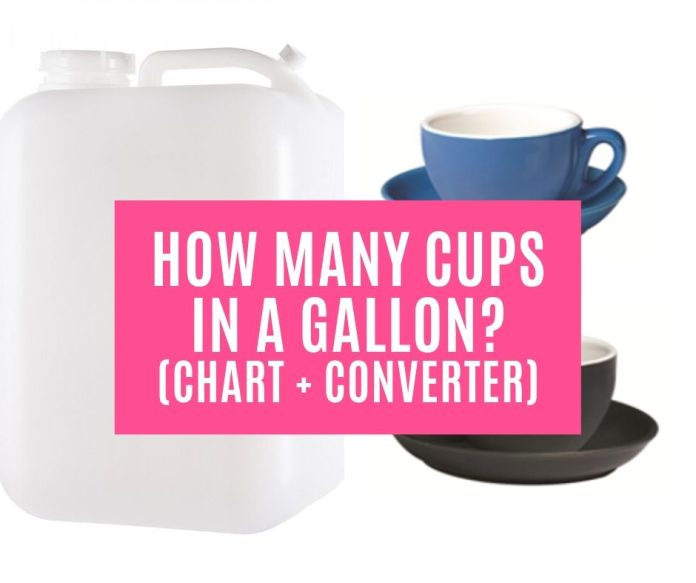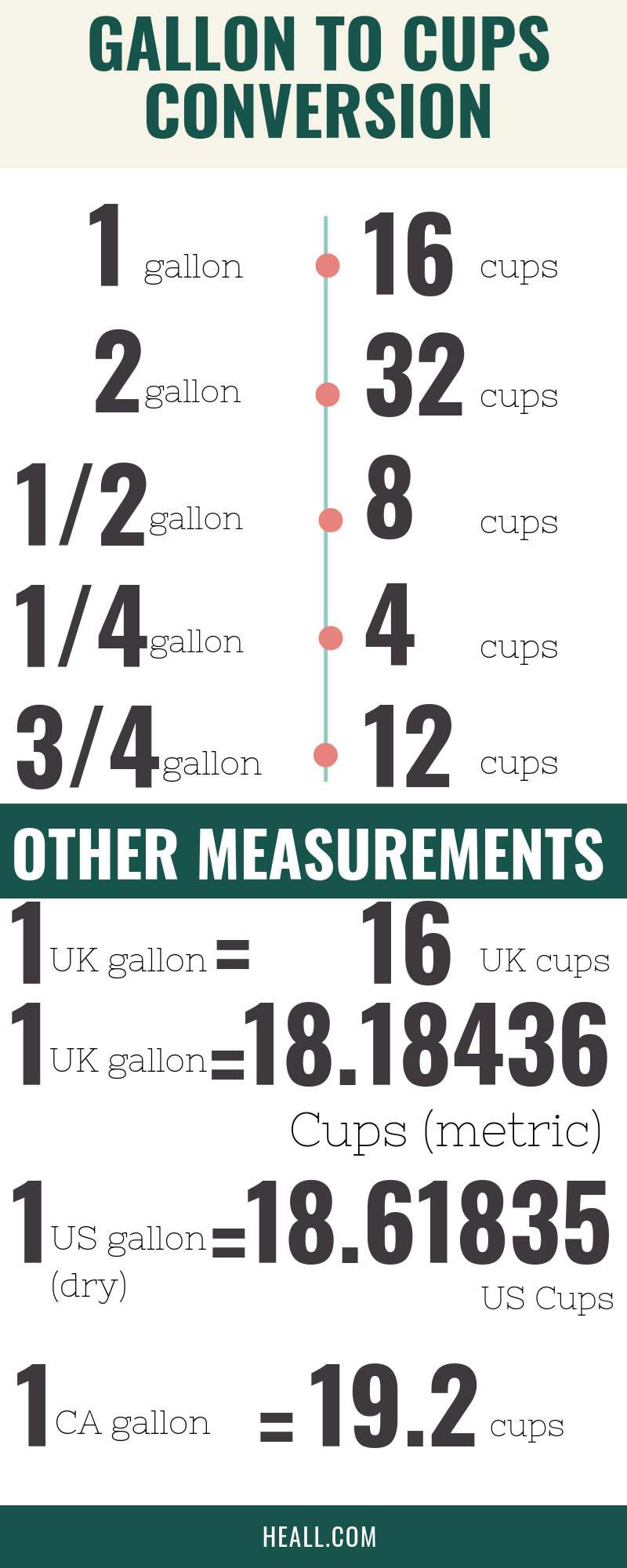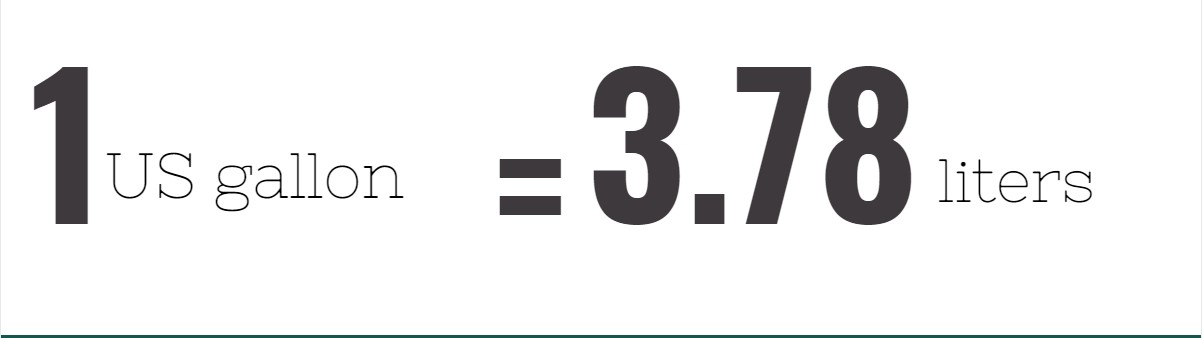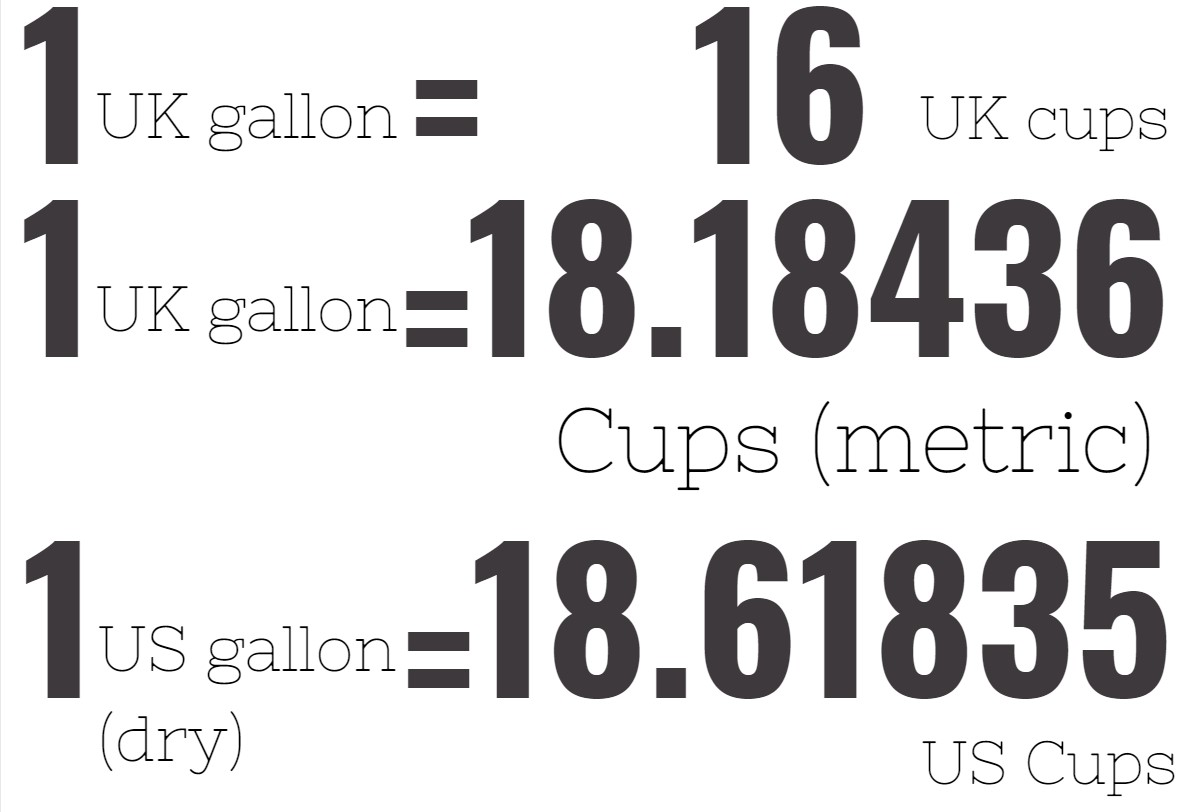# How Many Cups in a Gallon?

0
6933If we told you that our body needs at least half a gallon of water per day, would you believe this? A person who knows how many cups are in a gallon would easily tell it I both a yes and a no.

First of all, drinking cups are not standard measurements of cup to gallon because they vary in size. Second, what we are referring to here is the unit of measurement for volume called cup and how many cups are there in a gallon.

This is an important thing to know because cups and gallons are two of the most widely used units of measurements in serving sizes and of course in cooking. So back to the question. How many cups are in a gallon?

[CP_CALCULATED_FIELDS id=”9″]## What is US gallon?

Like the cup, gallon is also used as a unit of measurement for volume of both dry and liquid ingredients. Like all other unit conversions too, the gallon is measured in UK, imperial and US conversions. The US gallon when converted to liters would be 3.78.1 US gallon = 3.78 liters

According to Unit Converters, after the British colonial rule in the US, the US gallon was used particularly in measuring wine but evolved later on as a unit measurement for dry ingredients too like grains and corn, among others.

At present, the US gallon is an important unit of measurement in US supermarkets because it is used as the standard measurement for large containers of liquid products such as milk and even gasoline.

## Why is US gallon different to imperial gallon?

The US gallon should not be mistaken for the imperial gallon. In liters, the US gallon equates to 3.78L while the imperial gallon has 4.54L. In quarts, both gallon measurements equate to 4 quarts.

1 US gallon = 4 quarts = 3.78l

1 imperial gallon = 4 quarts = 4.54l

In 1824, the British monarchy adopted an imperial measurement for gallons which is based on 277.42 cubic inches of water while the US gallon was consistent with the 231 cubic inches of water. When expressed in cubic inches, we could see that the UK gallon is 20% greater in volume than the US gallon.

## How many cups in a gallon?

According to The Calculator Site, there are many ways to know how many cups are in a gallon. One US liquid gallon and one UK (imperial) gallon have the same conversion of 16 cups. The US dry gallon on the other hand is converted to 18.61 cups.1 US liquid gallon = 1 UK gallon = 16 cups

1 US dry gallon = 18.61 cups

But cups to dry gallons are no longer used in commercial settings because they are now using the unit of measurement called bushel for dry ingredients. For this matter, what you need to remember is that there are 16 cups in a gallon.

1 gallon = 16 cups

## How many 8 ounce cups are in a gallon?

This is a bit tricky because if you understand the question very well, it asks about the number of 8 ounces of that makes up a gallon. Good House Keeping reveals that there are 128 ounces in one gallon. From this, we divide 128 to 8 because we need to know the number of 8 ounce cups in a gallon. From these figures, we will get a value of 16. Thus, there are 16 8-ounce cups in a gallon.

1 gallon = 16 8-ounce cups

## How many cups in a gallon Canada?

According to Walley Central, Canada used the imperial metric system before the 1970s. After the 70s, they devised their own way of converting units of measurement. Thus, one Canadian gallon is equivalent to 1.2 US gallons.

Therefore, when we convert cups to gallons in Canada, we have to multiply 16 with 1.2 with 16 as the standard conversion for gallons to cups and 1.2 being the equivalent US gallon of Canadian gallon. From this conversion we get 19.2. Thus, there are 19.2 cups in a gallon in Canada. It is quite a misnomer therefore, that Canadian metric system is still the same as the imperial metric system.

1 CA gallon = 19.2 cups

## How many cups in a half gallon of milk?

Because we now know that there are 16 cups in a gallon, a half gallon milk would have 8 cups on it. We can arrive at this figure by multiplying 16 to 0.5. So the next time you chug milk, you would know how much of it you are drinking.

1 half gallon of milk = 8 cups

## How many 32 oz cups make a gallon?

In this section, we follow the same computation for the 8 ounce cups in a gallon. Since we know that there are 128 ounces in a gallon, we will divide 128 to 32.

We will get the value of 4. In this case, it is revealed that 32 ounce cups is equivalent to half a gallon. And because we also know that 32 ounces is equal to one quart, then there are also four quarts in one gallon.

1 gallon = 4 32-ounce cups

## How many cups of water in a gallon?

Regardless if you are using the imperial gallon or US liquid gallon, one gallon of water is composed of 16 cups. Take note that what is asked here is the number of cups in unit measurement to gallon and not the number of drinking cups composing a gallon. These are two different things.

1 gallon = 16 cups of water

## How to measure

To measure cups to gallons, you need the conversion standard of 16 cups per gallon. Since you know this, you will only have to multiply 16 to the number of gallons that you need. Of course, there are other ways for you to arrive at cup to gallon measurements.

1 gallon = 16*n cups (n: number of gallons)

Sometimes you need to convert to ounces first by multiplying or dividing 128 because that is the value of an ounce to gallon. Sometimes you also need to basic conversions from quarts and pints to gallons to cup to arrive at an accurate conversion of what you need.

## Cup to gallon chart

Conversion procedures of cups to gallons would be easier with the use of a conversion chart. For you have to have ease in doing so, refer to the cup to gallon chart below:

[CP_CALCULATED_FIELDS id=”10″]

CupsGallonCupsGallon
10.0625211.3125
20.125221.375
30.1875231.4375
40.25241.5
50.3125251.5625
60.375261.625
70.4375271.6875
80.5281.75
90.5625291.8125
100.625301.875
110.6875311.9375
120.75322
130.8125332.0625
140.875342.125
150.9375352.1875
161362.25
171.0625372.3125
181.125382.375
191.1875392.4375
201.25402.5

If you also need a gallon to cup chart measurement, you can refer to the chart below:

[CP_CALCULATED_FIELDS id=”9″]

GallonCupsGallonCups
11621336
23222352
34823368
46424384
58025400
69626416
711227432
812828448
914429464
1016030480
1117631496
1219232512
1320833528
1422434544
1524035560
1625636576
1727237592
1828838608
1930439624
2032040640

These are not hard conversions to remember. As a matter of fact, you only need to memorize the base conversion of cup to gallon which is 0.625 and gallon to cup at 16. When you know these base conversions, you will only have to multiply and divide them depending on what you need.

## Conclusion

In conclusion, cups to gallon conversions are essential specifically in measuring or converting liquid products and ingredients. We have mentioned in the beginning that both units of measurement are used to measure volume capacity.

Although each unit of measurement are categorized under either US cup or gallon and UK/imperial cup or gallon, the good thing about this conversion is that they follow the standard one gallon is equal to 16 cups ratio. The tricky part is actually converting cup to gallons because one cup is equivalent to 0.625 cups.

So why is this conversion an ease to know? Because it is functional. Be it for measuring your liquid ingredients or just monitoring your fluid intake, knowing how many cups in a gallon is an essential knowledge to have.